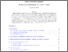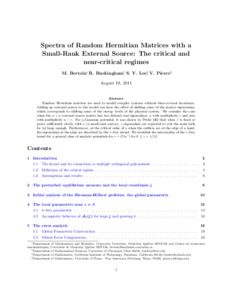Title:

# Spectra of Random Hermitian Matrices with a Small-Rank External Source: The Critical and Near-Critical Regimes

Bertola, Marco, Buckingham, R., Lee, S. Y. and Pierce, V. (2012) Spectra of Random Hermitian Matrices with a Small-Rank External Source: The Critical and Near-Critical Regimes. Journal of Statistical Physics, 146 (3). pp. 475-518. ISSN 0022-4715Preview Text (application/pdf) bertola2012b.pdf - Accepted Version 686kB

Official URL: http://dx.doi.org/10.1007/s10955-011-0409-2

## Abstract

Random Hermitian matrices are used to model complex systems without time-reversal invariance. Adding an external source to the model can have the effect of shifting some of the matrix eigenvalues, which corresponds to shifting some of the energy levels of the physical system. We consider the case when the n×n external source matrix has two distinct real eigenvalues: a with multiplicity r and zero with multiplicity n−r. For a Gaussian potential, it was shown by Péché (Probab. Theory Relat. Fields 134:127–173, 2006) that when r is fixed or grows sufficiently slowly with n (a small-rank source), r eigenvalues are expected to exit the main bulk for |a| large enough. Furthermore, at the critical value of a when the outliers are at the edge of a band, the eigenvalues at the edge are described by the r-Airy kernel. We establish the universality of the r-Airy kernel for a general class of analytic potentials for r=O(n γ ) for 0≤γ<1/12.

Divisions: Concordia University > Faculty of Arts and Science > Mathematics and Statistics Article Yes Bertola, Marco and Buckingham, R. and Lee, S. Y. and Pierce, V. Journal of Statistical Physics 2012 10.1007/s10955-011-0409-2 Riemann-Hilbert problem Asymptotic analysis Nonlinear steepest descent analysis r-Airy kernel Critical phenomena 976934 Danielle Dennie 05 Mar 2013 15:58 18 Jan 2018 17:43

## References:

Adler, M., Delépine, J., van Moerbeke, P.: Dyson’s nonintersecting Brownian motions with a few outliers. Commun. Pure Appl. Math. 62, 334–395 (2009) »

2. Adler, M., Orantin, N., van Moerbeke, P.: Universality of the Pearcey process. » arXiv:0901.4520 [math-ph] (2009)

3. Aptekarev, A., Bleher, P., Kuijlaars, A.: Large n limit of Gaussian random matrices with external source, part II. Commun. Math. Phys. 259, 367–389 (2005) »

4. Baik, J., Ben Arous, G., Péché, S.: Phase transition of the largest eigenvalue for non-null complex sample covariance matrices. Ann. Probab. 33, 1643–1697 (2005) »

5. Baik, J.: Painleve formulas of the limiting distributions for non-null complex sample covariance matrices. Duke Math. J. 133, 205–235 (2006) »

6. Baik, J.: On the Christoffel-Darboux kernel for random Hermitian matrices with external source. Comput. Methods Funct. Theory 9, 455–471 (2009). » arXiv:0809.3970 [math.CV] (2008)

7. Baik, J., Wang, D.: On the largest eigenvalue of a Hermitian random matrix model with spiked external source I. Rank one case. » arXiv:1010.4604 [math-ph] (2010)

8. Baik, J., Wang, D.: On the largest eigenvalue of a Hermitian random matrix model with spiked external source II. Higher rank case. » arXiv:1104.2915 [math-ph] (2011)

9. Bertola, M.: Biorthogonal polynomials for two-matrix models with semiclassical potentials. J. Approx. Theory 144, 162–212 (2007) »

10. Bertola, M., Buckingham, R., Lee, S.Y., Pierce, V.: Spectra of random Hermitian matrices with a small-rank external source: the supercritical and subcritical regimes. » arXiv:1009.3894 [math-ph] (2010)

11. Bertola, M., Lee, S.Y.: First colonization of a spectral outpost in random matrix theory. Constr. Approx. 30(2), 225–263 (2010) »

12. Bertola, M., Lee, S.Y.: First colonization of a hard-edge in random matrix theory. Constr. Approx. 31(2), 231–257 (2010) »

13. Bertola, M., Yo, M.Y.: Commuting difference operators, spinor bundles and the asymptotics of orthogonal polynomials with respect to varying complex weights. Adv. Math. 220, 154–218 (2009)

14. Bleher, P., Delvaux, S., Kuijlaars, A.: Random matrix model with external source and a constrained vector equilibrium problem. » arXiv:1001.1238 [math-ph] (2010)

15. Bleher, P., Kuijlaars, A.: Random matrices with external source and multiple orthogonal polynomials. Int. Math. Res. Not. 3, 109–129 (2004)

16. Bleher, P., Kuijlaars, A.: Large n limit of Gaussian random matrices with external source, part I. Commun. Math. Phys. 252, 43–76 (2004)

17. Bleher, P., Kuijlaars, A.: Large n limit of Gaussian random matrices with external source, part III: double scaling limit. Commun. Math. Phys. 270, 481–517 (2007) »

18. Brézin, E., Hikami, S.: Correlations of nearby levels induced by a random potential. Nucl. Phys. B 479, 697–706 (1996)

19. Brézin, E., Hikami, S.: Spectral form factor in a random matrix theory. Phys. Rev. E 55, 4067–4083 (1997) »

20. Brézin, E., Hikami, S.: Extension of level-spacing universality. Phys. Rev. E 56, 264–269 (1997)

21. Brézin, E., Hikami, S.: Level spacing of random matrices in an external source. Phys. Rev. E 58, 7176–7185 (1998)

22. Deift, P.: Orthogonal Polynomials and Random Matrices: A Riemann-Hilbert Approach. Am. Math. Soc., Providence (1998)

23. Deift, P., Kriecherbauer, T., McLaughlin, K.: New results on the equilibrium measure for logarithmic potentials in the presence of an external field. J. Approx. Theory 95, 388–475 (1998)

24. Deift, P., Kriecherbauer, T., McLaughlin, K., Venakides, S., Zhou, X.: Uniform asymptotics for polynomials orthogonal with respect to varying exponential weights and applications to universality questions in random matrix theory. Commun. Pure Appl. Math. 52, 1335–1425 (1999)

25. Deift, P., Kriecherbauer, T., McLaughlin, K., Venakides, S., Zhou, X.: Strong asymptotics of orthogonal polynomials with respect to exponential weights. Commun. Pure Appl. Math. 52, 1491–1552 (1999)

26. Ercolani, N., McLaughlin, K.: Asymptotics of the partition function for random matrices via Riemann-Hilbert techniques and applications to graphical enumeration. Int. Math. Res. Not. 14, 755–820 (2003)

27. Ince, E.: Ordinary Differential Equations. Dover, New York (1944)

28. Kuijlaars, A., McLaughlin, K.: Generic behavior of the density of states in random matrix theory and equilibrium problems in the presence of real analytic external fields. Commun. Pure Appl. Math. 53, 736–785 (2000)

29. McLaughlin, K.: Asymptotic analysis of random matrices with external source and a family of algebraic curves. Nonlinearity 20, 1547–1571 (2007)

30. Péché, S.: The largest eigenvalue of small rank perturbations of Hermitian random matrices. Probab. Theory Relat. Fields 134, 127–173 (2006)

31. Saff, E., Totik, V.: Logarithmic Potentials with External Fields. Springer, Berlin, (1997)

32. Tracy, C., Widom, H.: Level-spacing distributions and the Airy kernel. Commun. Math. Phys. 159, 151–174 (1994) »

33. Wigner, E.: On the statistical distribution of the widths and spacings of nuclear resonance levels. Proc. Camb. Philos. Soc. 47, 790–798 (1951)

34. Zinn-Justin, P.: Random Hermitian matrices in an external field. Nucl. Phys. B 497, 725–732 (1997)

35. Zinn-Justin, P.: Universality of correlation functions of Hermitian random matrices in an external field. Commun. Math. Phys. 194, 631–650 (1998)
All items in Spectrum are protected by copyright, with all rights reserved. The use of items is governed by Spectrum's terms of access.

Repository Staff Only: item control pageResearch related to the current document (at the CORE website)
Back to top